# Series- Test 1

1)

Find the missing figure in the series from the given answer figures.

[SSC CGL, 1999]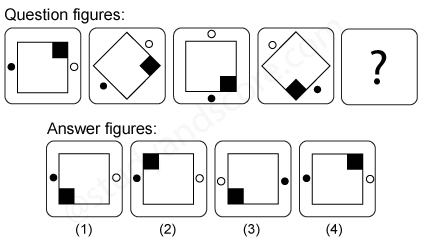2)

Find the missing figure in the series from the given answer figures.

[SSC CGL, 1999]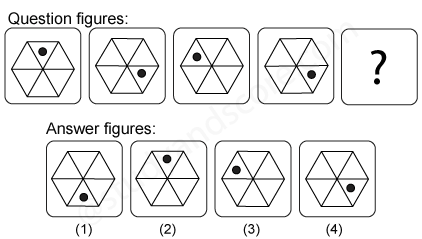3)

Find the missing figure in the series from the given answer figures.

[SSC CGL, 1999]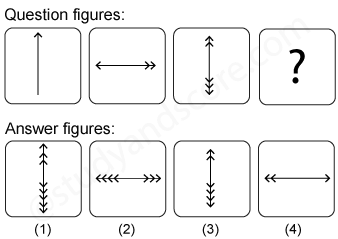4)

Find the missing figure in the series from the given answer figures.

[SSC CGL, 1999]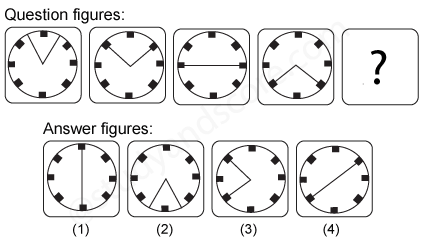5)

Complete the series by selecting the most appropriate answer figure.

[SSC CGL, 2000]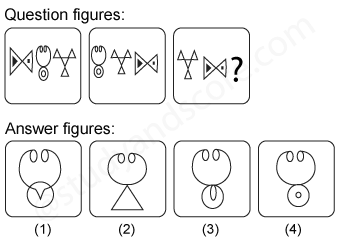--Share with your friends --